Courses

# Chemistry: CBSE Sample Question Paper (2020-21) - 3 Notes | EduRev

## Class 12 : Chemistry: CBSE Sample Question Paper (2020-21) - 3 Notes | EduRev

The document Chemistry: CBSE Sample Question Paper (2020-21) - 3 Notes | EduRev is a part of the Class 12 Course Sample Papers for Class 12 Medical and Non-Medical.
All you need of Class 12 at this link: Class 12

Class - XII
Chemistry
TIME: 3 Hrs.
M.M: 80

General Instructions:
(a) There are 33 questions in this question paper. All questions are compulsory.
(b) Section A: Q. No. 1 to 16 are objective type questions. Q. No. 1 and 2 are passage based questions carrying 4 marks each while Q. No. 3 to 16 carry 1 mark each. MCQs or Reason Assertion Type based on given passage each carrying 1 mark.
(c) Section B: Q. No. 17 to 25 are Short Answer Questions and carry 2 marks each.
(d) Section C: Q. No. 26 to 30 are Short Answer Questions and carry 3 marks each.
(e) Section D: Q. No. 31 to 33 are Long Answer Questions carrying 5 marks each.(f) There is no overall choice. However, internal choices have been provided.
(g) Use of calculators and log tables is not permitted.

Section - A

Objective Type

Q1. All real structures are three dimensional structures. They can be obtained by stacking two dimensional layers one above the other. While placing the second square close-packed layer above the first we follow the same rule that was followed when one row was placed adjacent to the other. The second layer is placed over the first layer such that the spheres of the upper layer are exactly above those of the first layer. In this arrangement spheres of both the layers are perfectly aligned horizontally as well as vertically.

A metallic element crystallises into a lattice having a ABC ABC ... pattern and packing of spheres leaves out voids in the lattice.

The following questions are multiple choice questions. Choose the most appropriate answer:

(i) What type of structure is formed by this arrangement ?
(a) ccp
(b) hcp
(c) ccp/fcc
(d) none of the above
Ans. c

(ii) Name the non-stoichiometric point defect responsible for colour in alkali metal halides
(a)
Frenkel defect
(b) Interstitial defect
(c) Schottky defect
(d) F-centres
Ans. d

(iii) What is the total volume of atoms in a face centred cubic unit cell of a metal ? (r is atomic radius)
(a)
16/3 πr3
(b) 8 πr3
(c) 8/3 πr3
(d) 1/3 πr3
Ans. a
Solution. Number of atoms per unit cell in fcc = 4
∴ Total volume of atoms present in fcc unit cell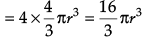(iv) Which of the following statements is not true for the amorphous and crystalline solids?
(a) Amorphous solids are isotropic and crystalline solids are anisotropic.
(b) Amorphous solids are short range order and crystalline solids are long range order.
(c) Amorphous solids melt at characteristic temperature while crystalline solids melt over a range of temperature.
(d) Amorphous solids have irregular shape and crystalline solids have a geometrical shape.
Ans. c
Solution. Crystalline solids melt at characteristic temperature while amorphous solids melt over a range of temperature.

OR
Which of the following statements is not true for unit cell?
(a) Each cubic unit cell has 8 atoms on its corners, the total number of atoms in one unit cell is 1.
(b) A unit cell is characterised by its dimensions along the three edges a,b,c.
(c) Each body centred cubic cell has 2 atoms in one unit cell.
(d) Each face centred cubic cell contains only one constituent particle present at the centre of each face.
Ans. d
Solution. Each fcc unit cell contains one constituent particle present at the centre of each face, besides the ones that are at its corners.

In transition elements, generally, ions of the same charge in a given series show progressive decrease in radius with increasing atomic number. This is because the new electron enters a d orbital each time the nuclear charge increases by unity. But the radii of the third (5d) series are virtually the same as those of the corresponding members of the second series. This phenomenon is associated with the intervention of the 4f orbitals which must be filled before the 5d series of elements begin. The filling of 4f before 5d orbital results in a regular decrease in atomic radii called Lanthanoid contraction.

In these questions, a statement of assertion followed by a statement of reason is given. Choose the correct answer out of the following choices.
(a) Assertion and reason both are correct statements and reason is correct explanation for assertion.
(b) Assertion and reason both are correct statements but reason is not correct explanation for assertion.
(c) Assertion is correct statement but reason is wrong statement.
(d) Assertion is wrong statement but reason is correct statement.
Following are the transition metal ions of 3d series : Ti4+, V2+, Mn3+, Cr3+
(Atomic number : Ti = 22, V = 23, Mn = 25, Cr = 24)
(i) Assertion : Among the given ions, Cr3+ is the most stable in an aqueous environment.
Reason : Cr3+ has half filled t32g.
Ans. d

(ii) Assertion : Among the given ions, Mn3+ is the most strong oxidizing agent.
Reason : Mn3+ has an unstable configuration.
Ans. c
Solution. Mn3+, due to stable d5 configuration in Mn2+

(iii) Assertion : Ti4+ ion is colourless.
Reason : All valence electrons are unpaired in Ti4+ ion.
Ans. a
Solution. Ti4+, No unpaired electrons

(iv) Assertion : Atomic radii of third series of transition elements is similar to that of the second series.
Reason : According to Aufbau’s principle 4f orbitals needs to be filled before the 5d series begin.

Following questions (No. 3-11) are Multiple Choice Questions carrying 1 mark each:
Q3. The correct order of increasing acidic strength is:
(a)
Phenol < Ethanol < Chloroacetic acid < Acetic acid
(b) Ethanol < Phenol < Chloroacetic acid < Acetic acid
(c) Ethanol < Phenol < Acetic acid < Chloroacetic acid
(d) Chloroacetic acid < Acetic acid < Phenol < Ethanol.
Ans. c
Solution. Phenol is more stable than alcohol due to formation of more stable conjugate base after removal of H+ from phenol.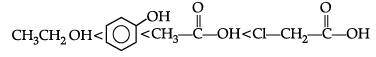Whereas, carboxylic acid is more acidic than acetic acid due to formation of more stable conjugate base after removal of H+ as compared to phenol.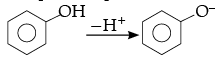Chloroacetic acid is more acidic than acetic acid due to the presence of electron withdrawing chlorine group attached to a- carbon of carboxylic acid.

OR
What kind of compounds undergo cannizaro reactions ?
(a)
Ketones with no a- hydrogen
(b) Aldehydes with a- hydrogen
(c) Carboxylic acids with a- hydrogen
(d) Aldehydes with no a- hydrogen
Ans. d
Solution. Aldehydes with no α- hydrogen undergo cannizaro reaction.

Q.4. Electrode potential for Mg electrode varies according to the equation:
(a)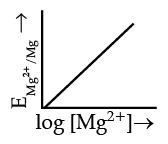(b)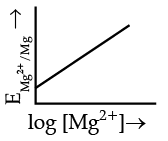(c)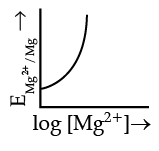(d)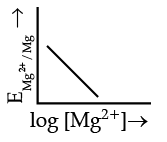Ans. b
Solution.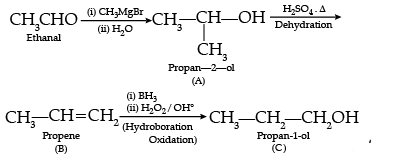OR
According to Arrhenius equation, rate constant k is equal to Ae–Ea/RT. Which of the following options represent the graph of ln k versus 1/T ?
(a)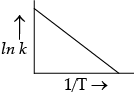(b)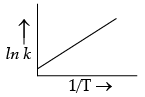(c)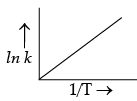(d)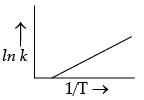Ans. a
Solution.
These linkages are present between 5' and 3' of pentose sugars of nucleotides.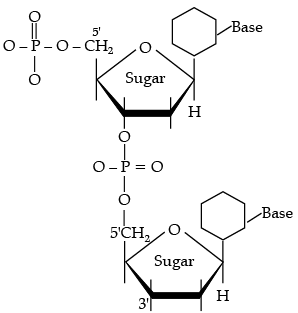Q.5. A first order reaction is 50% completed in 1.26 × 1014 s. How much time would it take for 100% completion?
(a)
1.26 × 1015 s
(b) 2.52 × 1014 s
(c) 2.52 × 1028 s
(d) Infinite
Ans. d
Solution. The reaction will be 100% complete only after infinite time.

Q.6. Generally, transition elements form coloured salts due to the presence of unpaired electrons. Which of the following compounds will be coloured in solid state ?
(a) Ag2SO4
(b) CuF2
(c) ZnF2
(d) Cu2Cl2
Ans. b
Solution. Cu2+ has 1 unpaired electron in CuF2 which is responsible for imparting colour in solid state.

Q.7. Consider a first order gas phase decomposition reaction given below:
A(g) → B(g) + C(g)
The initial pressure of the system before decomposition of A was ‘pi’. After lapse of time ‘t’, total pressure of the system increased by x units and became ‘pt’. The rate constant k for the reaction is given as:

(a)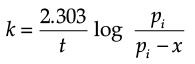(b)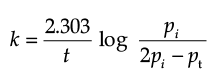(c)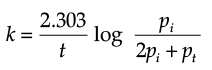(d)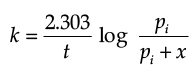Ans. b
Solution. Let us consider a first order gas phase decomposition reaction : A(g) ® B (g) + C(g) The initial pressure of the system before decomposition of A is ‘Pi.’ After lapse of time ‘t’, total pressure of the system increased by x units and became ‘Pt’.

Hence, the pressure of A decreased by x atom.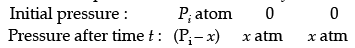Pt = (Pi – x) + x + x
= Pi + x atm.
x = Pt – Pi
= Pi – (P+ Pi)
PA = 2P– Pt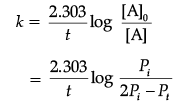Q.8. Which of the following is an iso-electronic pair ?
(a)
ICl2, ClO2
(b)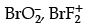(c) ClO2, BrF
(d) CN, O3
Ans. b
Solution.
(a) ICl2  = 53 + 2 × 17 = 87
ClO2 = 17 + 16 = 33
(b) BrO2− = 35 + 2 × 8 + 1 = 52
BrF2+ = 35 +9 × 2 − 1 = 52
(c) ClO2 = 17 + 16 = 33
BrF = 35 + 9 = 44
(d) CN = 6 + 7 + 1 = 14
O3 = 8 × 3 = 24

Q.9. Common name of Ethane-1,2-dioic acid is known as
(a)
Oxalic acid
(b) Phthalic acid
(d) Acetic acid
Ans. a
OR
Write the product(s) in the following reactions :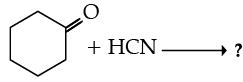(a) No product formed
(b)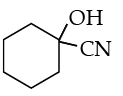(c)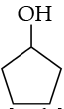(d)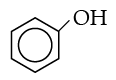Ans. b

Q.10. Using IUPAC norms write the formulae for the following :
Tris(ethane-1,2-diamine)chromium(III) chloride
(a) [Cr(NH2)2Cl]en3
(b) [Cr(en)3]Cl3
(c) [Cr(Cl)3]en3
(d) [Cr(NH2)2]en3Cl
Ans. b

OR
Using IUPAC norms write the formulae for the following :

(i) Sodium dicyanidoaurate (I)
(a) Na[Au(CN)2]
(b) Au[Na(CN)2]
(c) Na[(CN)2Au]
(d) None of the above
Ans. a

Q.11. What is the structural difference between starch and cellulose ?
(a)
In starch, glucose monomers are in α- helix configuration, while in cellulose, glucose monomers are in β-sheet configuration.
(b) In starch, glucose monomers are in β-configuration, while in cellulose, glucose monomers are in α- configuration.
(c) In starch, glucose monomers are in α- configuration, while in cellulose, glucose monomers are in β-configuration.
(d) None of the above.
Ans. c
Solution. In starch, glucose monomers are in α- configuration, while in cellulose, glucose monomers are in β-configuration.

OR
Glycogen is a branched chain polymer of α-D glucose units in which chain is formed by C1-C4 glycosidic linkage whereas branching occurs by the formation of C1-C6 glycosidic linkage. Structure of glycogen is similar to _____.
(a)
Amylose
(b) Amylopectin
(c) Cellulose
(d) Glucose
Ans. b
Solution. Structure of glycogen is similar to amylopectin. It is a branched chain polymer of α D glucose units in which chain is formed by  C1-C4 glycosidic linkage whereas branching occurs by the formation of C1-C6 glycosidic linkage.

In the following questions (Q. No. 12 - 16), a statement of assertion followed by a statement of reason is given.
Choose the correct answer out of the following choices.
(a) Assertion and reason both are correct statements and reason is correct explanation for assertion.
(b) Assertion and reason both are correct statements but reason is not correct explanation for assertion.
(c) Assertion is correct statement but reason is wrong statement.
(d) Assertion is wrong statement but reason is correct statement.

Q.12. Assertion (A) : Ecell should have a positive value for the cell to function.
Reason (R) : Ecathode < Eanode.
Ans. c
Solution. Ecell = Ecathode – Eanode.
To have positive value of Ecell, Ecathode should be greater than Eanode.

OR
Assertion (A) : Λm for weak electrolytes shows a sharp increase when the electrolytic solution is diluted.
Reason (R) : For weak electrolytes degree of dissociation increases with dilution of solution.
Ans. a
Solution. Weak electrolytes dissociate partially in concentrated solution. On dilution, their degree of dissociation increases hence, their Λm increases sharply.

Q.13. Assertion (A) : D(+)– Glucose is dextrorotatory in nature.
Reason (R) : ‘D’ represents its dextrorotatory nature.
Ans. c
Solution. 'D' corresponds to the position of –OH group on the right side on the farthest asymmetric C-atom.

Q.14. Assertion (A) : Cu cannot liberate hydrogen from acids.
Reason (R) : Because it has positive electrode potential.
Ans. a
Solution. Copper (Cu) does not liberate hydrogen from acids due to the presence of positive electrode potential.

Q.15. Assertion (A) : N-Ethylbenzene sulphonamide is soluble in alkali.
Reason (R) : Hydrogen attached to nitrogen in sulphonamide is strongly acidic.
Ans. c
Solution. In alkylation, an amine can react with alkyl halide to form next higher class of amine caused by the presence of electron pair on nitrogen which makes amine to behave as nucleophile and alkyl halide thus undergo nucleophilic substitution reaction. When primary and secondary amines react with acid chlorides, anhydrides and esters to give monosubstituted amides as products. Acylation is carried out in the presence of a base stronger than the amine like pyridine which causes the shift of the equilibrium to the right side.

Q.16. Assertion (A) : Cu is less reactive than hydrogen.
Reason (R) : EºCu2+/Cu is negative.
Ans. c
Solution. Cu is less reactive than hydrogen because EΘCu2+/cu  is positive.

Section - B

The following questions, Q.No 17 – 25 are Short Answer Type-I and carry 2 marks each.
Q.17. Account for the following:
(i)
O2N – CH2 – COOH has lower pKa value than CH3COOH.
(ii) (CH3)2CH – CHO undergoes aldol condensation whereas (CH3)3C – CHO does not.
Ans. (i) Due to electron withdrawing nature of –NO2 group which increases the acidic strength and decreases the pKa value.
(ii) (CH3)2CH-CHO has one a-H atom whereas α -H atom is absent in (CH3)3C-CHO.

(ii) Aldol condensation occurs due to presence of a-hydrogen. As (CH3)2CH–CHO has one a-H atom whereas (CH3)3C-CHO does not have a-H atom. Thus, (CH3)2CH–CHO undergoes aldol condensation whereas (CH3)3C–CHO does not.

OR
Write chemical equations for the following reactions:
(i)  Propanone is treated with dilute Ba(OH)2.
(ii) Acetophenone is treated with Zn(Hg)/ Conc. HCl.
Ans.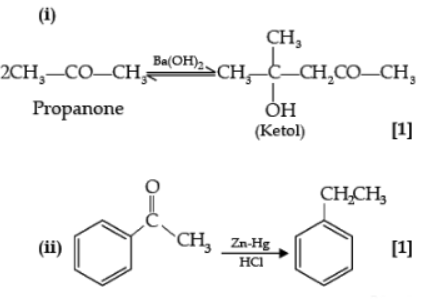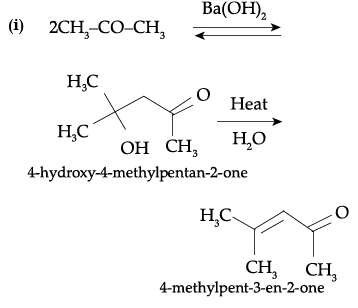Q.18. Write the equations involved in the following reactions :
(i)
Wolff-Kishner reduction
(ii) Etard reaction
Ans. (i) Wolff-Kishner reduction: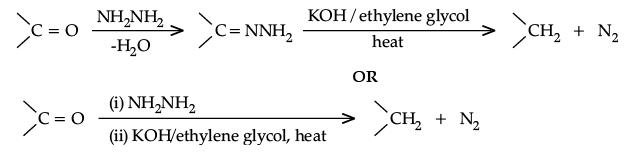(ii) Etard reaction: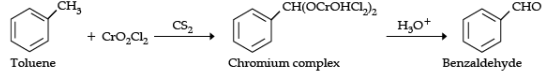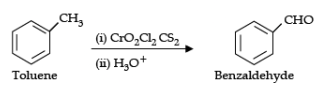OR
Write the reactions involved in the following:
(i)
Hell-Volhard-Zelinsky reaction
(ii) Decarboxylation reaction
Ans.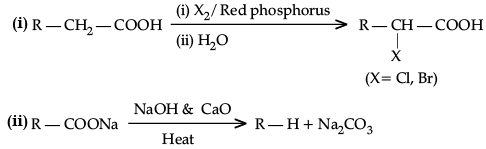Q.19. (i) Glucose on reaction with acetic acid gives glucose penta acetate. What does it suggest about the structure of glucose ?
(ii) Write difference between fibrous protein and globular protein. Give two points.

Ans. (i) 5 OH groups are present.
(ii) Fibrous proteins : parallel polypeptide chain, insoluble in water, Globular proteins : spherical shape, soluble in water, (or any 1 suitable difference)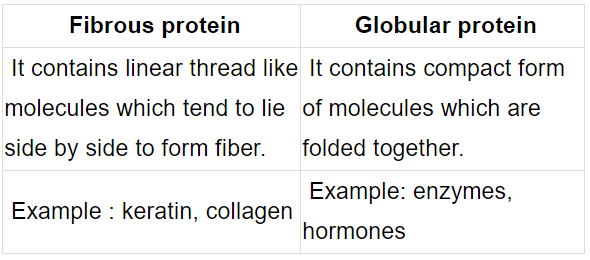OR
(i) What is the difference between a nucleoside and nucleotide ?
(ii) Write one difference between a-helix and b-pleated sheet structures of protein.
Ans.
(i) Nucleoside: It is formed when pentose sugar combines with nitrogen base.

Nucleotide : When nucleoside bonds with phosphate group
(ii) a-helix has intramolecular hydrogen bonding while β-pleated has intermolecular hydrogen bonding / a-helix results due to regular coiling of polypeptide chains while in β-pleated all polypeptide chains are stretched and arranged side by side.

Q.20. Give reasons for the following :
(i) (CH3)3 P = O exists but (CH3)3 N = O does not.
(ii) Oxygen has less electron gain enthalpy with negative sign than sulphur.
Ans.
(i) Because as N can't form 5 covalent bonds as its maximum covalency is three. The octet cannot be extended as it doesn't have d orbital, while P can extend its octet as it has empty d orbital.
(ii) This is due to very small size of oxygen atom, repulsion between electrons is large in relatively small 2p sub-shell.

Q.21. Calculate the emf of the following cell at 298 K
Cr(s)/Cr3+(0.1M)//Fe2+ (0.01M)/Fe(s)
[Given : E°cell = + 0.30 V]

Ans. 2Cr(s) + 3 Fe2+(aq.) → 3Fe(s) + 2Cr3+ (aq.)
n = 6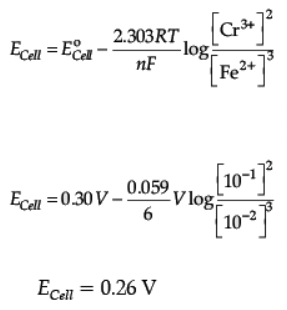OR
The conductivity of 10-3 mol/L acetic acid at 25°C is 4.1 × 10–5 S cm–1. Calculate its degree of dissociation if ∧0m for acetic acid at 25°C is 390.5 S cm2 mol-1.

Ans.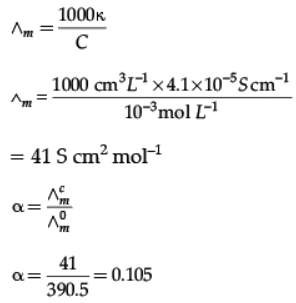Q.22. Complete the following equations :
(i)
Ag + PCl5 ®
(ii) CaF2 + H2SO4 ®
Ans. (i) 2Ag + PCl5 ® 2AgCl + PCl3
(ii) CaF2 + H2SO4 ® CaSO4(s) + 2HF (or H2F2)

Q.23. A black compound of manganese reacts with a halogen acid to give greenish yellow gas. When excess of this gas reacts with NH3 an unstable tri-halide is formed. How does the oxidation state of nitrogen changes in this process ? Identify the greenish yellow gas.
Ans.
The oxidation state of the nitrogen changes from –3 to +3 in the given reaction. Greenish yellow gas is chlorine.

MnO2 is the black color compound of manganese which reacts with halogen acid like HCl, to form greenish yellow chlorine gas. Cl2 in turn reacts with NH3 to form NCl3 where the oxidation state of N in NH3 is changed from –3 to +3 in NCl3.
MnO2 + 4HCl → MnCl2 + 2H2O + Cl2.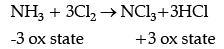Q.24. A reaction is first order in A and second order in B
(i)
Write the differential rate equation.
(ii) How is the rate affected on increasing the concentration of B three times ?
(iii) How is the rate affected when the concentration of both A and B are doubled ?
Ans. (i) Rate = k [H2O2] [ I]
(ii) Order = 2
(iii) Step 1

(i) The rate law for the reaction is :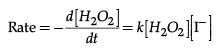(ii) This reaction is first order with respect to both H2O2 and I.
The overall order of the reaction is bimolecular, 2. The order of the reaction is determined from the slowest step of the reaction mechanism.
(iii) The first reaction is slow, so this is the rate determining step.

Q.25. Complete and balance the following chemical equations :
(a)
Fe2+ + MnO4 + H+ ®
(b) MnO4 + H2O + I– ®
Ans.
(a) 5Fe2++ MnO4 + 8H+ ® Mn2+ + 4H2O + 5Fe3+
(b) 2MnO4 + H2O + I ® 2MnO2 + 2OH + IO3

Section - C

Q.No 26 -30 are Short Answer Type II carrying 3 marks each.
Q.26. The vapour pressure of pure liquids A and B at 400 K are 450 and 700 mm Hg respectively. Find out the composition of liquid mixture if total pressure at this temperature is 600 mm Hg.
Ans.
ptotal = p1o + (p2o - p1o)k2
600 = 450 + (700 - 450)k2
k2 = 0.6
k1 =  1 - 0.6 = 0.4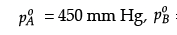= 450 mm Hg
pTotal = 600 mm Hg
According to Raoult’s law,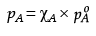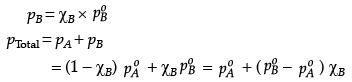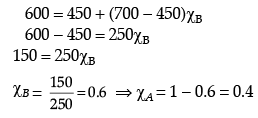OR
Using Raoult’s law explain how the total vapour pressure over the solution is related to mole fraction of components in the following solutions:
(i) CHCl3(l) and CH2Cl2(l)
(ii) NaCl(s) and H2O(l)
Ans. (i)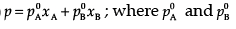are the vapour pressures of pure components (A) and (B) while xand xB are the mole fractions of the components (A) and(B) in the solution.
(ii) NaCl(s) is a non-volatile solute. When it is dissolved in water, the vapour pressure is lowered. Vapour pressure of solution can be calculated using the following relations:
p = p0xA
Where, xA = mole fraction of solvent
p0 = vapour pressure of pure solvent
p = vapour pressure of solution
Similarly,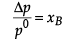Δp = (p0 - p) xB = mole fraction of solute.

Q.27. (i) Solutions of two electrolytes ‘A’ and ‘B’ are diluted. The limiting molar conductivity of ‘B’ increases 1.5 times while that of ‘A’ increases 25 times. Which of the two is a strong electrolyte ? Justify your answer.
(ii) The products of electrolysis of aqueous NaCl at the respective electrodes are :
Cathode : H2
Anode : Cl2 and not O2. Explain.
Ans. (i) ‘B’ is a strong electrolyte.
A strong electrolyte is already dissociated into ions, but on dilution interionic forces overcome, ions are free to move. So there is slight increase in molar conductivity on dilution.
(ii) On anode, water should get oxidised in preference to Cl-, but due to overvoltage/ overpotential Cl- is oxidised in preference to water.

Q.28. How will you convert the following :
(i) Nitrobenzene into aniline,
(ii) Ethanoic acid into methanamine,(iii) Aniline to N-phenylethanamide. (Write the chemical equations involved.)
Ans.
(i)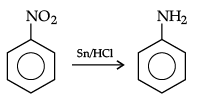(ii)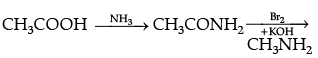(iii)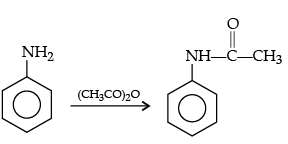OR
Give the structure of A, B and C in the following reactions :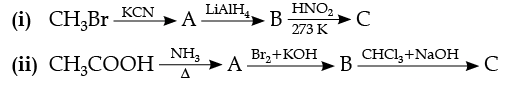Ans.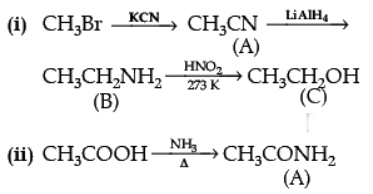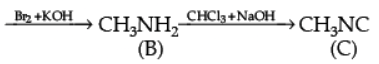Q.29. The rate of a reaction depends upon the temperature and is quantitatively expressed as:
(i) If a graph is plotted between log k and 1/T, write the expression for the slope of the reaction ?
(ii) If at under different conditions, Ea1 and Ea2 are the activation energy of two reactions. If Ea1 = 40J/ mol and Ea2 = 80 J/mol. Which of the two has a larger value of the rate constant ?
(iii) Write units of rate constants for zero order and second order reactions if the concentration is expressed in mol L-1 and time in seconds.
Ans.
(i) Slope = Ea/2.303R
(ii) k1 > k2
(iii) Zero order : mol L–1 s–1
Second order : L mol–1 s–1

(ii)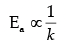So, as the activation energy (Ea) increases, the value of rate constant (k) decreases.

Hence, k1 > k2 > as E1 < E2
(iii) Zero order : mol L–1 s–1
Second order : L mol–1 s–1

Q.30. Write the structures of A, B and C in the following :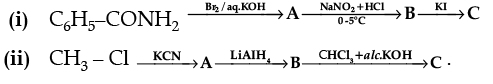Ans.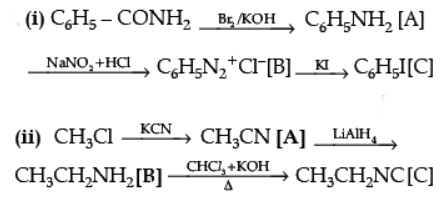Section - D

Q.No 31 to 33 are Long Answer Type carrying 5 marks each.
Q.31. Discuss briefly giving an example in each case the role of coordination compounds in :

(a) Biological system.
(b) Medicinal chemistry.
(c) Analytical chemistry.
(d) Extraction/metallurgy of metals.

Ans. (a) R ole of coordination compounds in biological systems : We know that photosynthesis is made possible by the presence of the chlorophyll pigment. This pigment is a coordination compound of magnesium. In the human biological system, several coordination compounds play important roles. For example, the oxygen-carrier of blood (haemoglobin) is a coordination compound of iron.
(b) Role of coordination compounds in medicinal chemistry : Certain coordination compounds of platinum (e.g., cis-platin) are used for inhibiting the growth of tumours.
(c) Role of coordination compounds in analytical chemistry : During salt analysis, a number of basic radicals are detected with the help of the colour changes they exhibit with different reagents. These colour changes are a result of the coordination compounds or complexes that the basic radicals form with different ligands.
(d) Role of coordination compounds in extraction or metallurgy of metals : The process of extraction of some of the metals from their ores involves the formation of complexes. For example, in aqueous solution, gold combines with cyanide ions to form [Au(CN)2]. From this solution, gold is later extracted by the addition of zinc metal.

OR
(a) When a co-ordination compound CoCl3.6NH3 is mixed with AgNO3, 3 moles of AgCl are precipitated per mole of the compound. Write
(i) Structural formula of the complex,
(ii) IUPAC name of the complex.

(b) Write the IUPAC name of the following :
(i) [Co(NH3)6]Cl3
(ii) [NiCl4]2–
(iii) K3[Fe(CN)6]

Ans.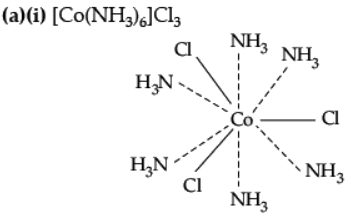(ii) IUPAC name : Hexamminecobalt (III) chloride.
(b)(i) Hexaamminecobalt (III) chloride.
(ii) Tetrachloridonickelate (II) ion.
(iii) Potassiumhexacyanoferrate (III)

Q.32. Write the main product(s) in each of the following reactions :
(i)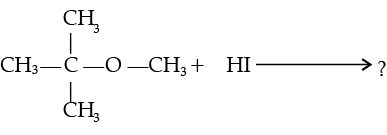(ii)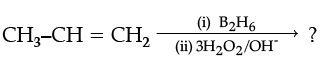(iii)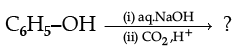(iv) Write the IUPAC name of the following compound: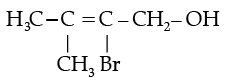(v) Write the IUPAC name of the following compound :
C6H5–CH2–CH2–OH

Ans.
(i)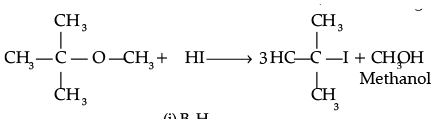(ii)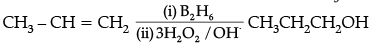(iii)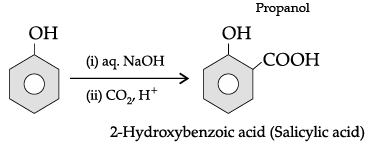(iv) 2-Bromo-3-methylbut-2-en-1-ol.
(v) Phenylethanol.

OR
(i) Name and complete the following chemical reaction :
RCH2COOH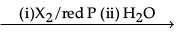(ii) Draw the structures of the following derivatives :
(a) The 2, 4-Dinitrophenylhydrazone of benzaldehyde,
(b) Acetaldehyde dimethyl acetal
(c) Cyclopropanone oxime.
Ans.
(i)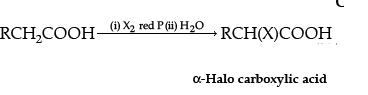The name of the reaction is Hell-Volhard-Zelinsky reaction.
(ii)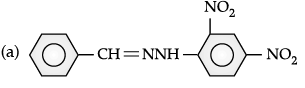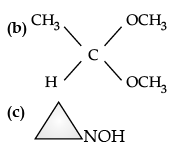Q.33. (A), (B) and (C) are three non-cyclic functional isomers of a carbonyl compound with molecular formula C4H8O. Isomers (A) and (C) give positive Tollens’ test whereas isomer (B) does not give Tollens’ test but gives positive Iodoform test. Isomers (A) and (B) on reduction with Zn(Hg)/conc. HCl give the same product (D).
(a)
Write the structures of (A), (B), (C) and (D).
(b)
Out of (A), (B) and (C) isomers, which one is least reactive towards addition of HCN ?
Ans.
Isomer (B) 2-butanone is least reactive towards addition of HCN. Towards addition reaction, aldehydes are more reactive than ketones due to steric and electronic reasons.
A nucleophile can approach more easily to aldehyde carbonyl carbon than that of ketone as in aldehydes, only one alkyl group is present and in ketones two bulky alkyl groups are present. Due to +I effect of alkyl groups, the carbonyl carbon in aldehydes is more electrophilic than that in ketones. In ketones +I effect of two alkyl groups reduces the electrophilicity of carbonyl carbon. In aldehyde, +I effect of only alkyl group reduces the electrophilicity of carbonyl carbon.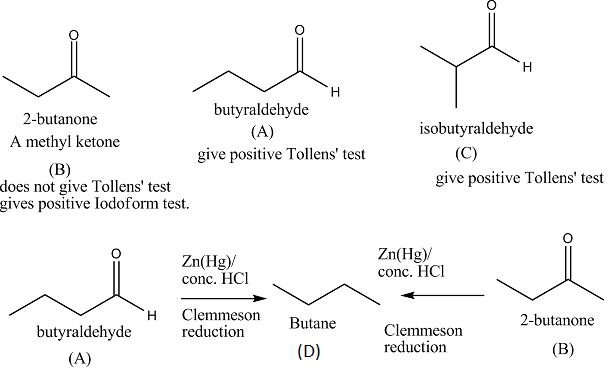OR
(i) Give reasons for the following :
(a) Protonation of Phenols is difficult whereas ethanol easily undergoes protonation.
(b) Boiling point of ethanol is higher than that of dimethyl ether.
(c) Anisole on reaction with HI gives phenol and CH3– I as main products and not iodobenzene and CH3OH.

(ii) Write the mechanism of the following reaction :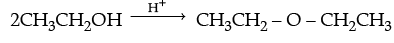Ans. (i) (a) In phenol, the lone pair of electrons on oxygen involves in delocalization which results in their non-availability for the protonation. Whereas in ethanol, the electrons on oxygen atom are not delocalised which results in their availability for protonation.
(b) The variation can be established by intermolecular hydrogen bonding, where alcoholic hydrogen is bounded to a strongly electronegative oxygen atom to give rise to

dipole of the form, Et–Oδ- – Hδ+ which arises the boiling point.

Whereas, C-O bonds in dimethyl ether are polar. Carbon bonded with hydrogen is moderately electronegative compared to oxygen. In the absence of intermolecular hydrogen bonding, ether becomes more volatile.
(c) Attack of I–ion on anisole is through SN2 mechanism, which attacks the lesser hindered CH3 group forming CH3I. Also, C-O bond in ether is difficult to break due to resonance.

(ii)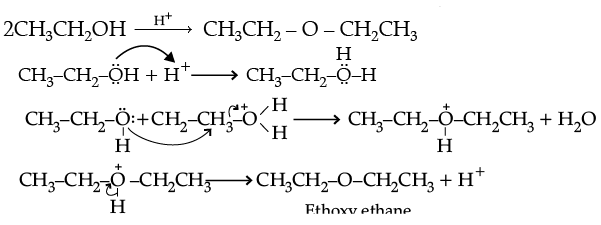Offer running on EduRev: Apply code STAYHOME200 to get INR 200 off on our premium plan EduRev Infinity!

67 docs

,

,

,

,

,

,

,

,

,

,

,

,

,

,

,

,

,

,

,

,

,

;